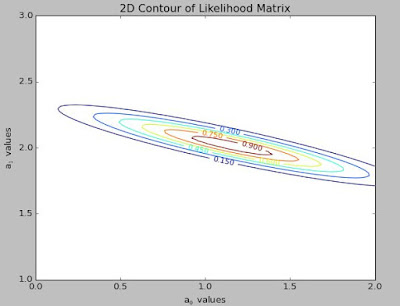## Tuesday, June 30, 2015

Another day, another blog.  Today in my very interactive Astro-statistics-Python-coding class, I gained a better understanding about the concepts of probability density/distribution function and the cumulative probability distribution function.  I will explain how the other interns and I have made significantly more progress in enhancing our Python scripts to do much more analysis regarding our use of simulated data that constitutes a linear trend with random noise added to it.  If you want to follow along, here is my code that you can copy and paste into a script or go to RAW and then download (with the "wget" Terminal command).  The next series of plots are but one of the many possible outcomes from executing my code:

>>>execfile('stat_ex_uncertainties')
>>>cdf(a_vals, plot=True)      # this can be ran however many times you wishFigure 1: Once again, 5 "x" values were chosen and a linear equation $$a_0 + a_1\times x_{vals} +$$ noise$$_{random}$$  was used to simulate 5 measurements that were taken.  For this step, I chose the intercept and slope to be $$a_0=1$$ and $$a_1=2$$.Figure 2: Contour plot of the Likelihood matrix with the dimensions  (number of $$a_1$$ elements) X (number of $$a_0$$ elements).  The regions within the darker and redder lines indicate areas where the likelihood is maximized.  In this example, it seems to imply that $$a_1$$ values close to 2.0 paired with $$a_0$$ values near 1.0 are most likely to be the correct parameter values.  This is great!  The Likelihood-$$\chi^2$$ fitting was applied to the simulated data and it is determining what the two fitting parameters ($$a_0$$ and $$a_1$$) are.
A quick aside: why does the contour plot have that slope to it?
If you run my "cdf(a_vals, plot=True)" function multiple times, the values and eccentricities of the regions may change but it will still have that tilt.  As I am writing this blog, I force myself to explain these details meticulously, which in turn compels me to consider all questions that might arise in the thoughts of my audience.  The question I have posed is one I am still contemplating.  As of now, I think that the slope of the ellipses attests to how well the two parameters are being constrained.  The linear slope parameter $$a_1$$ is being constrained more than the intercept $$a_0$$.  I am inclined to believe that this is why the regions are stretched horizontally more than they are stretched vertically.

The next two plots reiterate and confirm what the contour plot is showing but from a 1-dimensional point of view.  (Thus the need for 2 plots to express the results of the 2D contour.)Figure 3: Marginalization of Likelihood for $$a_0$$.  In my Likelihood matrix, the columns are considered to be the x-axis ($$a_0$$) for the contour plot.  The rows act as the y-axis ($$a_1$$) for the contour plot.  Therefore, when I get the sum for each column of the matrix I obtain the summation of all $$a_0$$ likelihoods for each column.  Each of these sums have been plotted here.  The maximum marginalized likelihood seems to occur when $$a_0$$ is ~1.25.  This suggests that the best value for the $$a_0$$ fitting parameter is near 1.25, but I want to find a very precise number with minimal uncertainty for my fitting parameter.Figure 4: Marginalization of Likelihood for $$a_1$$.  The sum of each row in my likelihood matrix was taken and plotted here with the rows' corresponding value of $$a_1$$.  The maximum marginalized likelihood seems to occur when $$a_1$$ is equivalent to 2.0. In other words, this plot is stating that the correct value for $$a_1$$ is most likely 2.0.

Now that you're all caught up to speed, I can discuss the extra steps (since my last post) we have done for this exercise.  I guess you can call this Phase III just to stay consistent with the previous couple posts.

Figures 3 and 4 illustrate the Probability Density Function (PDF) that we know to be a Gaussian.  This means that the contour plot of Figure 2 yields a Gaussian distribution as well.  The data in those plots also show the best, or most satisfactory, value for each fitting parameter.  However, one of those circular data points may not reveal the most satisfactory value for the fitting parameter despite the fact that one of those points truly does look like it is at the peak of the curve (in both plots).  To find a value that is truly at the peak of the curve, I had to first integrate the PDF produced by my contour plot in Figure 2 and subsequently interpolate the resultant curve in order to find the x-value that corresponds to where the area under the curves of Figures 3 and 4 equate to $$\frac{1}{2}$$ the total area.

to be continued...